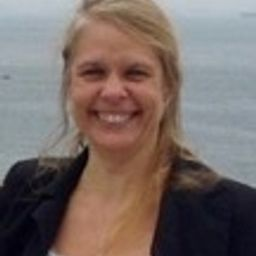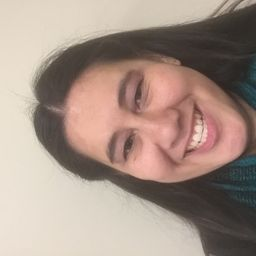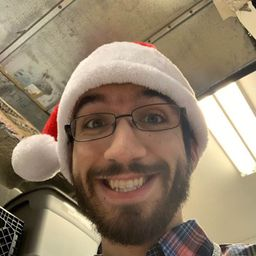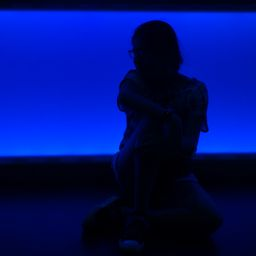# Geometry A Common Core Curriculum

## EducatorsCC
MY+ 2 more educators

### Problem 1

A number that describes the likelihood of an event is the ________ the event.Catt H.

### Problem 2

Describe the difference between theoretical probability and experimental probability.Amrita B.

### Problem 3

Find the number of possible outcomes in the sample space. Then list the possible outcomes.

You roll a die and flip three coins.

Check back soon!

### Problem 4

Find the number of possible outcomes in the sample space. Then list the possible outcomes.

You flip a coin and draw a marble at random from a bag containing two purple marbles and one white marble.Amrita B.

### Problem 5

Find the number of possible outcomes in the sample space. Then list the possible outcomes.

A bag contains four red cards numbered 1 through 4, four white cards numbered 1 through 4, and four black cards numbered 1 through 4. You choose a card at random.

Check back soon!

### Problem 6

Find the number of possible outcomes in the sample space. Then list the possible outcomes.

You draw two marbles without replacement from a bag containing three green marbles and four black marbles.Amrita B.

### Problem 7

A game show airs on television five days per week. Each day, a prize is randomly placed behind one of two doors. The contestant wins the prize by selecting the correct door. What is the probability that exactly two of the five contestants win a prize during a week? (See Example 2.)

CC
Charles C.

### Problem 8

Your friend has two standard decks of 52 playing cards and asks you to randomly draw one card from each deck. What is the probability that you will draw two spades?Amrita B.

### Problem 9

When two six-sided dice are rolled, there are 36 possible outcomes. Find the probability that (a) the sum is not 4 and (b) the sum is greater than 5 . (See Example 3.)

Check back soon!

### Problem 10

The age distribution of a population is shown. Find the probability of each event.

(Diagram Cant Copy)

a. A person chosen at random is at least 15 years old.
b. A person chosen at random is from 25 to 44 years old.Amrita B.

### Problem 11

A student randomly guesses the answers to two true-false questions. Describe and correct the error in finding the probability of the student guessing both answers correctly.

The student can either guess two incorrect answers, two correct answers, or one of each. So the probability of guessing both answers correctly is $\frac{1}{3}$ .

MY
Michael Y.

### Problem 12

A student randomly draws a number between 1 and $30 .$ Describe and correct the error in finding the probability that the number drawn is greater than 4 .

The probability that the number is less than 4 is $\frac{3}{30},$ or $\frac{1}{10}$ So , the probability that the number is greater than 4 is $1-\frac{1}{10}$ , or $\frac{9}{10}$ .Amrita B.

### Problem 13

You throw a dart at the board shown. Your dart is equally likely to hit any point inside the square board. What is the probability your dart lands in the yellow region? (See Example 4.)

CC
Charles C.

### Problem 14

The map shows the length (in miles) of shoreline along the Gulf of Mexico for each state that borders the body of water. What is the probability that a ship coming ashore at a random point in the Gulf of Mexico lands in the given state?
a. Texas
b. Alabama
c. Florida
d. LouisianaAmrita B.

### Problem 15

You roll a six-sided die 60 times. The table shows the results. For which number is the experimental probability of rolling the number the same as the theoretical probability? (See Example 5.)

(Table Cant Copy)

CC
Charles C.

### Problem 16

A bag contains 5 marbles that are each a different color. A marble is drawn, its color is recorded, and then the marble is placed back in the bag. This process is repeated until 30 marbles have been drawn. The table shows the results. For which marble is the experimental probability of drawing the marble the same as the theoretical probability?

(Table Cant Copy)Amrita B.

### Problem 17

Refer to the spinner shown. The spinner is divided into sections with the same area.
a. What is the theoretical probability that the spinner stops on a multiple of 3?
b. You spin the spinner 30 times. It stops on a multiple of 3 twenty times. What is the experimental probability of stopping on a multiple of 3?
c. Explain why the probability you found in part (b) is different than the probability you found in part (a).

Check back soon!

### Problem 18

Describe a real-life event that has a probability of 0. Then describe a real-life event that has a probability of 1.Amrita B.

### Problem 19

A survey of 2237 adults ages 18 and over asked which sport is their favorite. The results are shown in the figure. What is the probability that an adult chosen at random prefers auto racing? (See Example 6.)

(Figure cant Copy)

CC
Charles C.

### Problem 20

A survey of 2392 adults ages 18 and over asked what type of food they would be most likely to choose at a restaurant. The results are shown in the figure. What is the probability that an adult chosen at random prefers Italian food?

(Figure Cant Copy)Amrita B.

### Problem 21

Refer to the board in Exercise 13 . Order the likelihoods that the dart lands in the given region from least likely to most likely.
A. green
B. not blue
C. red
D. not yellow

Check back soon!

### Problem 22

Refer to the chart below. Order the following events from least likely to most likely.

(Chart Cant Copy)

A. It rains on Sunday.
B. It does not rain on Saturday.
C. It rains on Monday.
D. It does not rain on Friday.Amrita B.

### Problem 23

Use the figure in Example 3 to answer each question.
a. List the possible sums that result from rolling two six-sided dice.
b. Find the theoretical probability of rolling each sum.
c. The table below shows a simulation of rolling two six-sided dice three times. Use a random number generator to simulate rolling two six-sided dice 50 times. Compare the experimental probabilities of rolling each sum with the theoretical probabilities.

$$\begin{array}{|c|c|c|}\hline & {A} & {B} & {C} \\ \hline 1 & {\text { First Die }} & {\text { Second Die}} & {\text { Sum}} \\ \hline 2 & {4} & {6} & {10} \\ \hline 3 & {3} & {5} & {8} \\ \hline 4 & {1} & {6} & {7} \\ \hline\end{array}$$

Check back soon!

### Problem 24

You flip a coin three times. It lands on heads twice and on tails once. Your friend concludes that the theoretical probability of the coin landing heads up is $P(\text { heads up })=\frac{2}{3}$ . Is your friend correct? Explain your reasoning.Amrita B.

### Problem 25

A sphere fits inside a cube so that it touches each side, as shown. What is the probability a point chosen at random inside the cube is also inside the sphere?

Check back soon!

### Problem 26

Consider the graph of $f$ shown. What is the probability that the graph of $y=f(x)+c$ intersects the $x$ -axis when $c$ is a randomly chosen integer from 1 to 6? Explain.

(Graph Cant Copy)Amrita B.

### Problem 27

A manufacturer tests 1200 computers and finds that 9 of them have defects. Find the probability that a computer chosen at random has a defect. Predict the number of computers with defects in a shipment of $15,000$ computers. Explain your reasoning.Shana P.

### Problem 28

The tree diagram shows a sample space. Write a probability problem that can be represented by the sample space. Then write the answer(s) to the problem.

(Diagram Cant Copy)Amrita B.

### Problem 29

$$\frac{2 x^{3}}{x^{2}}$$

Check back soon!

### Problem 30

$$\frac{2 x y}{8 y^{2}}$$Amrita B.

### Problem 31

$$\frac{4 x^{9} y}{3 x^{3} y}$$Isaiah Q.

### Problem 32

$$\frac{6 y^{0}}{3 x^{-6}}$$Amrita B.

### Problem 33

$$(3 p q)^{4}$$Rujula D.
$$\left(\frac{y^{2}}{x}\right)^{-2}$$